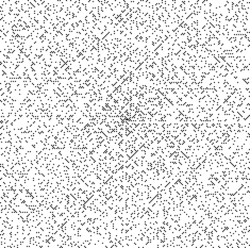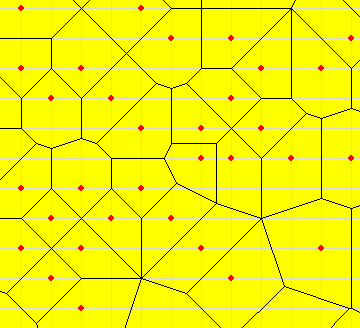# Voronoi Diagrams of the Ulam Prime Spiral

In 1963, the mathematician Stanislaw Ulam was sitting, bored, in a lecture. He started doodling on a piece of paper, drawing the numbers 1, 2, 3 and so on in a spiral shape.Then, he began to circle the prime numbers.This showed some remarkable, and as yet unexplained, patterns. The pictrue below shows each prime as a black dot, against the white background of non-primes.These images, by the way, are all from the Wikipedia Article on the Ulam Prime Spiral.

One day, I was sitting, bored, in my office. I started doodling on my computer screen, clicking the primes of the Prime Spiral into the DotPlacer Applet elsewhere on this site. I told the applet to draw the Voronoi Diagram of the Ulam Prime Spiral, and obtained the picture below. (By the way, I made a mistake. Can you spot it?)Then, I wrote a program to calculate a whole bunch of information about the Voronoi diagram. I've included some of this information on this site. Given a set of special points in the plane, each cell of the voronoi diagram contains exactly one of the special points. It also contains all points of the plane that are closer to that special point than to any other. The cells are therefore polygons (if finite) or infinite 'polygonal' regions - bounded by straight lines. For example, if the special points are fast food stores, the cells might give some idea of the 'reach' of each store - assuming people always buy their fast food at the nearest store.

Some of my thoughts regarding the Voronoi Diagram of the Ulam Prime Spiral :

• Since each 'special point' has integer coordinates, the corners of the voronoi diagram should all have rational coordinates. Therefore each cell has rational area, and its perimeter should be a sum of square roots of rationals.
• There should be no limit to the size of the cells - since primes become more and more rare as numbers get larger and larger.
• Each cell should be a finite polygon.
• If there exaists a cell of a particular shape, there should be infinitely many cells of that shape.
Note that some of these conjectures would be very hard to prove.

In any case, I've provided a number of HTML files, with tables of information about the cells in the Prime Spiral Voronoi Diagram. Each table gives a list of prime numbers, one number per row. For each prime, I give

• Its (x,y) coordinates in the Ulam Prime Spiral.
• The number of edges of its Voronoi cell.
• The actual coordinates of the corners of its Voronoi cell
• The area and perimeter of its cell (in exact form).

So what tables do I have?

• Firstly, you can see the cells for all primes less than 100 and less than 1000.
• Absolutely fascinating, yes? Well, I've also collected information about the smallest prime for which the cell is a triangle, quadrilateral, pentagon, hexagon, and so on, up to decagon. So if you're curious to see when the first n-gons appear, you can. Or maybe I'll tell you : these special primes are 313, 23, 2, 5, 61, 67, 2837 and 8741. There were no 11-gonal voronoi cells for primes less than 10000. However, I conjecture that eventually there should be an n-gonal voronoi cell for any n, no matter how large.
• Which voronoi cell has the largest area? I predict that there's no such thing - that as you explore further and further out, you'll keep on finding bigger and bigger voronoi cells. I've found out which primes have voronoi cells larger than any smaller prime - in terms of area (the winning primes are 2, 3, 5, 11, 23, 37, 47, 53, 79, 83 ...) and perimeter (2, 3, 5, 11, 23, 47, 79, 83, ...). Notice some similarities there? Not surprising. Generally speaking, as the perimeter goes up, so does the area, and vice-versa. In fact, the only primes in my 'perimeter' list that are not in my 'area' list are 337 and 7433 (but I only checked up to 10000).
• Some cells are round, others are long and thin. I measured the 'roundness' of each cell by dividing the perimeter squared by four times the area. For a circle, this would give Pi. For a square, 4. For a Voronoi cell? Well, you can see the cells with record-breaking roundness here - either more and more round or less and less. Amazingly, there's no prime less than 10242 with a 'rounder' voronoi cell than 67!
• A lot of primes have voronoi cells with integral area. Here they are
• There are far fewer with integral perimeter. Amongst the primes less than 10000, only 6829, 8539 and 9281 have integral perimeter. This page shows all those primes for which the voronoi cell's perimeter is a square root of a rational number (this includes rational perimeters too). Note that all of these cells are in fact rectangles! When I noticed that, I wondered why. I concluded that it's not that integer perimeter voronoi cells must be rectangles. Rather, that's just the easiest way for a voronoi cell to have this property. In fact, I managed to find a triangular voronoi cell with perimeter 36 and area 54. I explain how on this page.
Well, that's all for now. let me know about your own explorations of the voronoi diagram of the Ulam prime spiral!